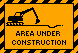# Programs & Data

### Demo programs for the benchmark DSGE emerging economy simulations: the monetary policy with a dualistic labor market paper

1. sosfindemo: program for model structure [matlab file]
2. sosfinbegdemo: program for initial conditions and output [matlab file]

### Demo programs for sustainable debt and deficits in emerging markets paper

1. sosdebt: program for model structure [matlab file]
2. sosdebtbeg: program for initial conditions and output [matlab file]

### Matlab subroutines called

1. Explanatory notes by Soderlind [PDF]
2. DiscAlg: Solves the LQ problem under discretion, iterating backwards in time [matlab file]
3. DiscAlg2.m [matlab file]
4. Var1SimPs: To calculate impulse response functions [matlab file]